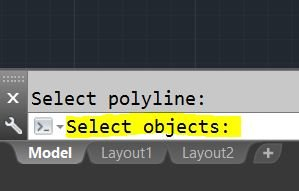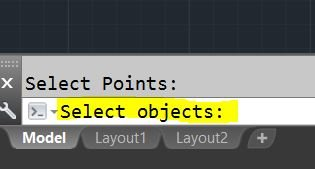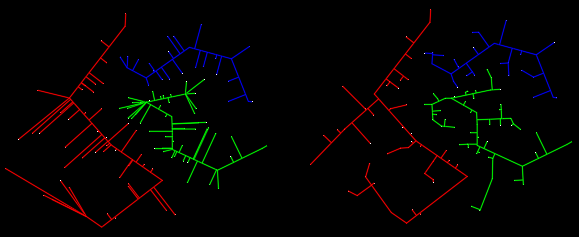Perpendicular Lines from many points to a Polyline

Recommended PostsHi, I'm a newbie to autocad LISP and also to the forum. I have to create perpendicular lines to a polyline from points adjoining to it. I can manually do that one by one but I have several thousand points either side of the very long polyline and it is difficult to do that one by one. Is there anyway i can quickly draw perpendicular lines from the points to the polyline. the drawing is 2D and below is a small sample to of the drawing just to illustrate.Share on other sitesMy code used to do a similar thing (you can find it in the list of my replies on this forum): move blocks perperdicular to multiple polylines, first find the closest polyline to the block.

I changed it to select points, and to draw lines instead of moving the blocks.  I think it does what you require,

but it has remnants of the old code, so it has lines of code that are not needed anymore.

So if you select multiple polylines it will draw a line to the closest one.

- Command STO (feel free to change this)

- select polyline(s)

-select points

```

(defun Line (p1 p2)
(entmakex (list (cons 0 "LINE")
(cons 10 p1)
(cons 11 p2)
)))

;;; Modified code from: http://www.arch-pub.com/Move-point-perpendicular-to-line_10272335.html
;;; By: Mel Franks

(defun c:sto ( / en obj pts_ss ss_len c pten ptobj pted pt pt2 this_is_the_point best_distance)
(princ "\nSelect polyline: ")
(setq en (ssget (list
(cons 0 "ARC,CIRCLE,ELLIPSE,LINE,LWPOLYLINE,POLYLINE,SPLINE")
))
)
(princ "\nSelect Points: ")
(setq pts_ss (ssget (list (cons 0 "POINT"))))
(setq ss_len (sslength pts_ss))
(setq c 0)
(while (< c ss_len)
(setq pten (ssname pts_ss c))
(setq ptobj (vlax-ename->vla-object pten))
(setq pted (entget pten))
(setq pt (cdr (assoc 10 pted)))

(setq cnt 0)
(setq best_distance 1000000)  ;; and we will search for something better.  As long as it keeps dropping we are happy
(setq this_is_the_point nil)
(while (< cnt (sslength en))
(setq ename (ssname en cnt))
(setq pt2 (vlax-curve-getClosestPointTo ename pt))
(if (< (distance pt pt2) best_distance) (progn
(setq best_distance (distance pt pt2))
(setq this_is_the_point pt2)
))
(setq cnt (+ cnt 1))
)
;;(vla-move ptobj (vlax-3d-point pt) (vlax-3d-point this_is_the_point))
(Line pt this_is_the_point)
;;(vla-Rotate ptobj (vlax-3d-point this_is_the_point) (angle pt this_is_the_point))
;;(vla-Rotate ptobj this_is_the_point 1 )
(setq c (+ c 1))
)
(princ)
)

```

•1

Share on other sitesDear Emmanuel Delay, Thank you very much. The code work very well and it does what I want.

Just one thing to ask, the text display on the command line always says "Select Object" in both instances (i.e. ask to select 'polyline' and 'points'). see below images. Is it possible to change to 'Select polyline' and 'Select Point'?

Thanks again.Share on other sitesYes, for example like this:

```
...

(setvar "nomutt" 1)  ;; shut up ssget
(setq en (ssget (list
(cons 0 "ARC,CIRCLE,ELLIPSE,LINE,LWPOLYLINE,POLYLINE,SPLINE")
))
)
(princ "\nSelect Points: ")

(setq pts_ss (ssget (list (cons 0 "POINT"))))
(setq ss_len (sslength pts_ss))
(setvar "nomutt" 0)  ;; set the variable back

...
```

•1

Share on other sitesHere's another -

```(defun c:ppl ( / ent idx pnt sel )
(if
(and (setq sel (LM:ssget "\nSelect points: " '(((0 . "POINT")))))
(progn
(while
(progn (setvar 'errno 0) (setq ent (entsel "\nSelect curve: "))
(cond
(   (= 7 (getvar 'errno))
(princ "\nMissed, try again.")
)
(   (null ent) nil)
(   (vl-catch-all-error-p (vl-catch-all-apply 'vlax-curve-getclosestpointto ent))
(princ "\nInvalid object selected.")
)
)
)
)
(setq ent (car ent))
)
)
(repeat (setq idx (sslength sel))
(setq idx (1- idx)
pnt (assoc 10 (entget (ssname sel idx)))
)
(entmake (list '(0 . "LINE") pnt (cons 11 (vlax-curve-getclosestpointto ent (cdr pnt)))))
)
)
(princ)
)

;; ssget  -  Lee Mac
;; A wrapper for the ssget function to permit the use of a custom selection prompt
;; msg - [str] selection prompt
;; arg - [lst] list of ssget arguments

(defun LM:ssget ( msg arg / sel )
(princ msg)
(setvar 'nomutt 1)
(setq sel (vl-catch-all-apply 'ssget arg))
(setvar 'nomutt 0)
(if (not (vl-catch-all-error-p sel)) sel)
)

Edited by Lee Mac
•2

Share on other sitesAnother one for fun```(defun c:foo (/ _dxf _sl a b c e p s x)
;; RJP » 2019-01-10
(defun _sl (s) (cond ((= 'pickset (type s)) (vl-remove-if 'listp (mapcar 'cadr (ssnamex s))))))
(defun _dxf (c e) (cdr (assoc c (entget e))))
(cond
((setq s (_sl (ssget '((0 . "LWPOLYLINE,INSERT,POINT,CIRCLE")))))
(foreach x	s
(if (= (_dxf 0 x) "LWPOLYLINE")
(setq a (cons x a))
(setq b (cons (_dxf 10 x) b))
)
)
(and a
b
(foreach p b
(setq c
(car	(vl-sort
(mapcar
'(lambda (x)
(list (setq c (vlax-curve-getclosestpointto x p)) (distance p c) (_dxf 8 x))
)
a
)
)
)
)
(setq e (entmakex (list '(0 . "line") (cons 10 p) (cons 11 (car c)) (cons 8 (caddr c)))))
;; This line below creates the right example comment out to get left
(setq a (cons e a))
)
)
)
)
(princ)
)```

Edited by ronjonp
•1

Share on other sitesThank you all..Join the conversation

You can post now and register later. If you have an account, sign in now to post with your account.
Note: Your post will require moderator approval before it will be visible.×   Pasted as rich text.   Paste as plain text instead

Only 75 emoji are allowed.